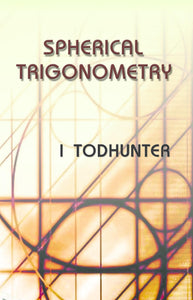# Spherical Trigonometry

Vendor

MJPPUB

Type

Books

Categories
200 in stock.
Quantity must be 1 or more
Regular price Rs. 160.00

Spherical Trigonometry

(For the use of Colleges and Schools with Numerous Examples)

Contents:

1. Great and Small Circles, 2. Spherical Triangles, 3. Spherical Geometry, 4. Relations between the Trigonometrical Functions of the Sides and the Angles of a Spherical Triangle, 5. Solution of Right-angled Triangles, 6. Solution of Oblique-angled Triangles, 7. Circumscribed and Inscribed Circles, 8. Area of a Spherical Triangle Spherical Excess, 9. On Certain Approximate Formulae, 10. Geodetical Operations, 11. On Small Variations in the Parts of a Spherical Triangle, 12. On the Connexion of Formulae in Plane and Spherical Trigonometry, 13. Polyhedrons, 14. Arcs Drawn to Fixed Points on the Surface of a Sphere, 15. Miscellaneous Propositions, 16. Numerical Solution of Spherical Triangles.

Sale

Unavailable

Sold Out